CBSE Class 10 Sample Paper for 2018 Boards

Class 10
Solutions of Sample Papers for Class 10 Boards

### A train travelling at a uniform speed for 360 km would have taken 48 minutes less to travel the same distance if its speed were 5 km/hour more. Find the original speed of the train.

This is a question of CBSE Sample Paper - Class 10 - 2017/18.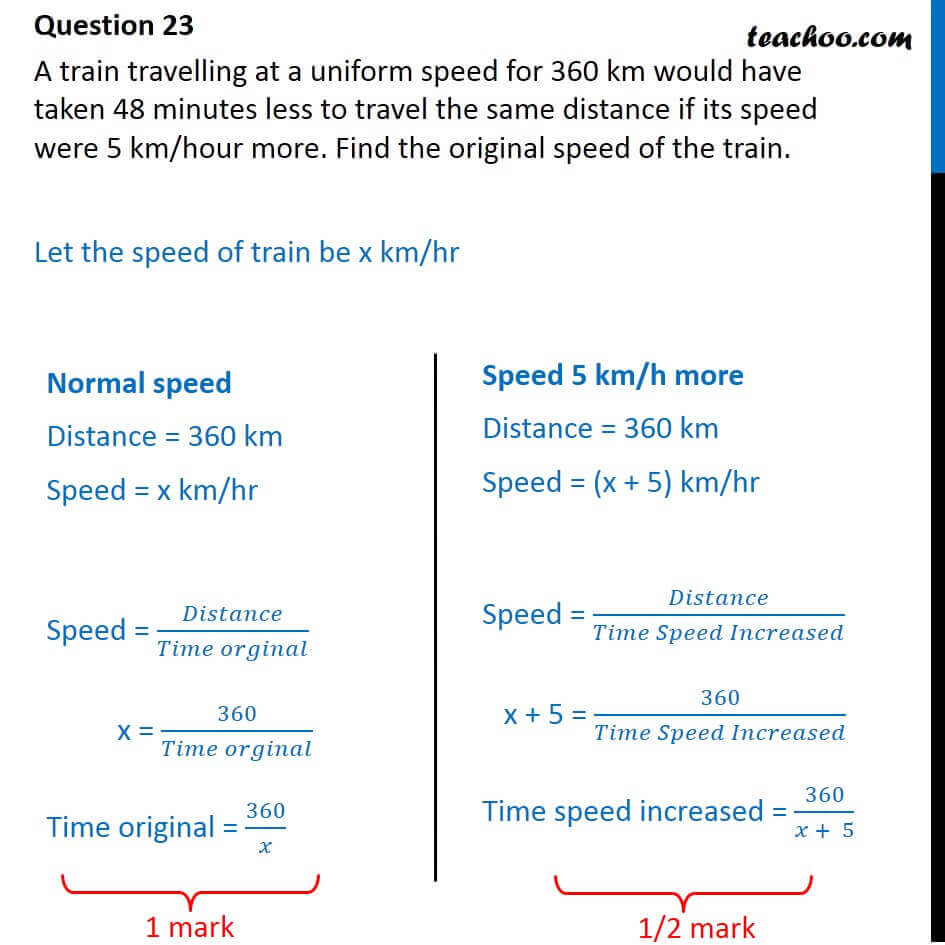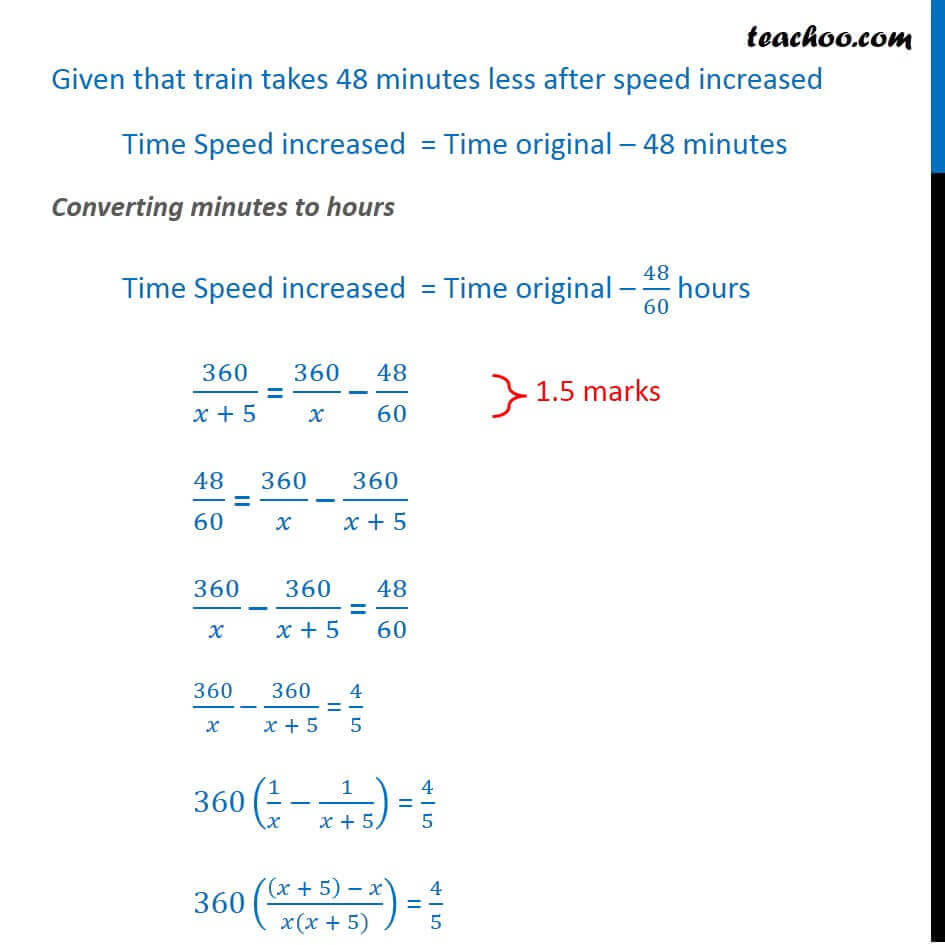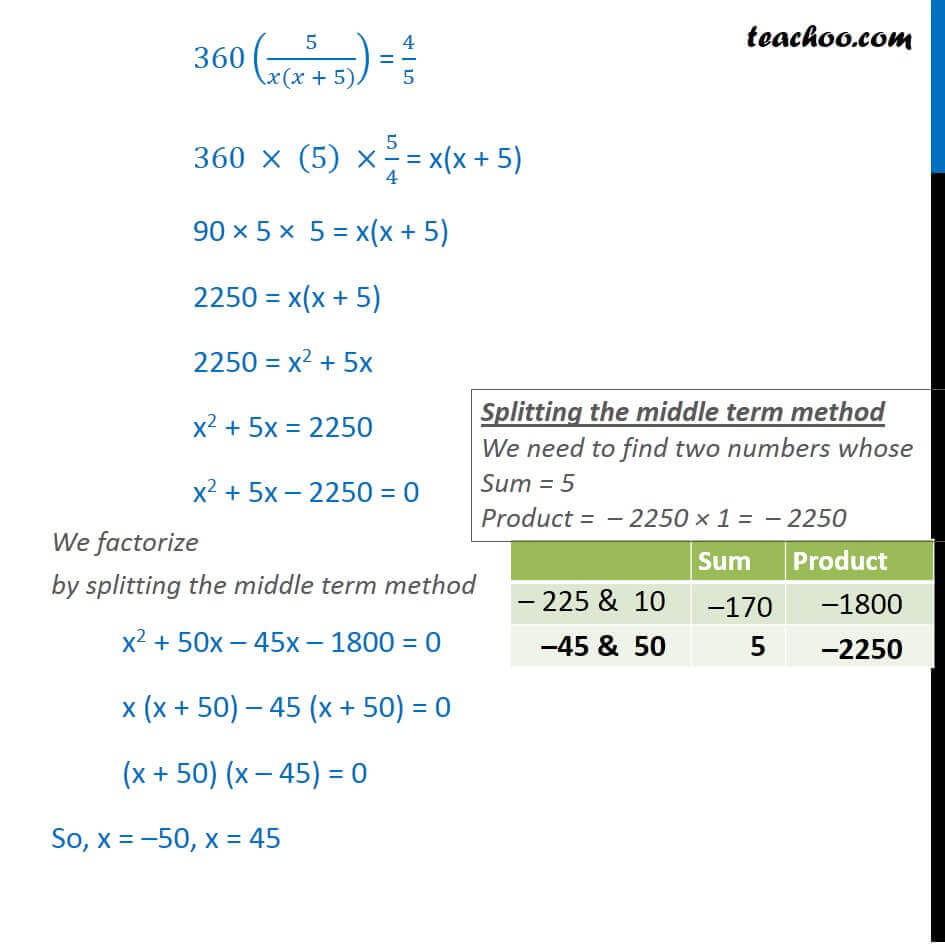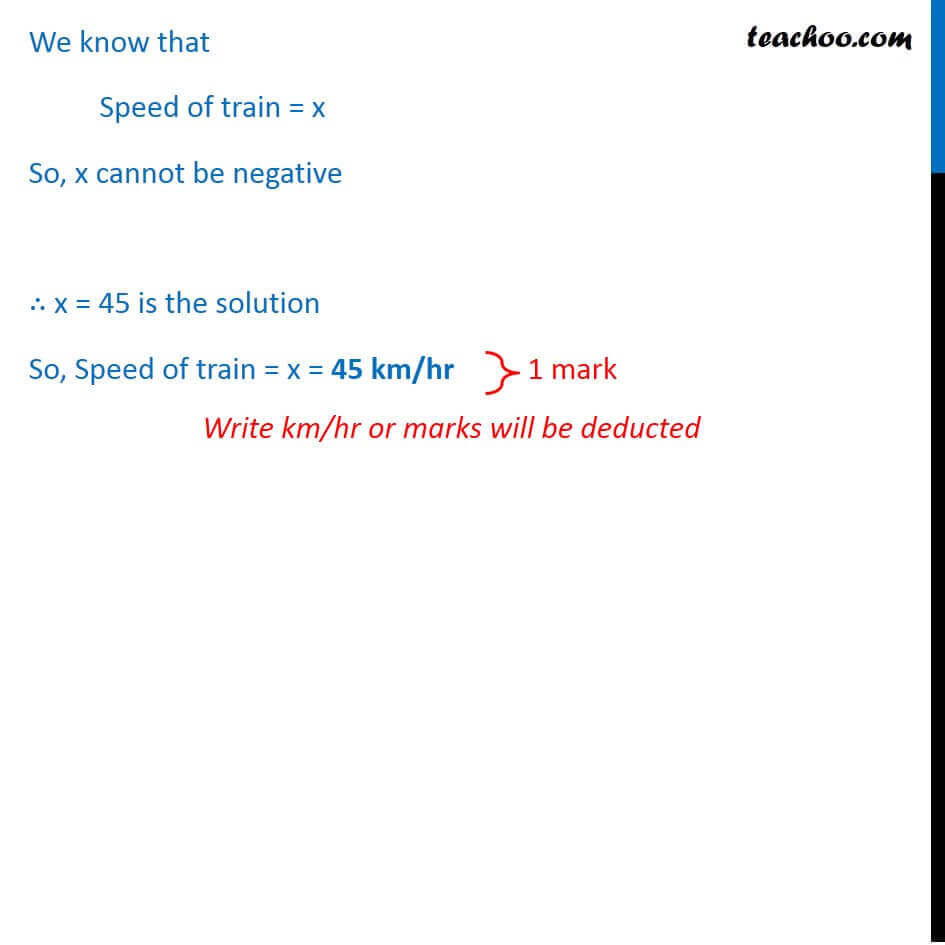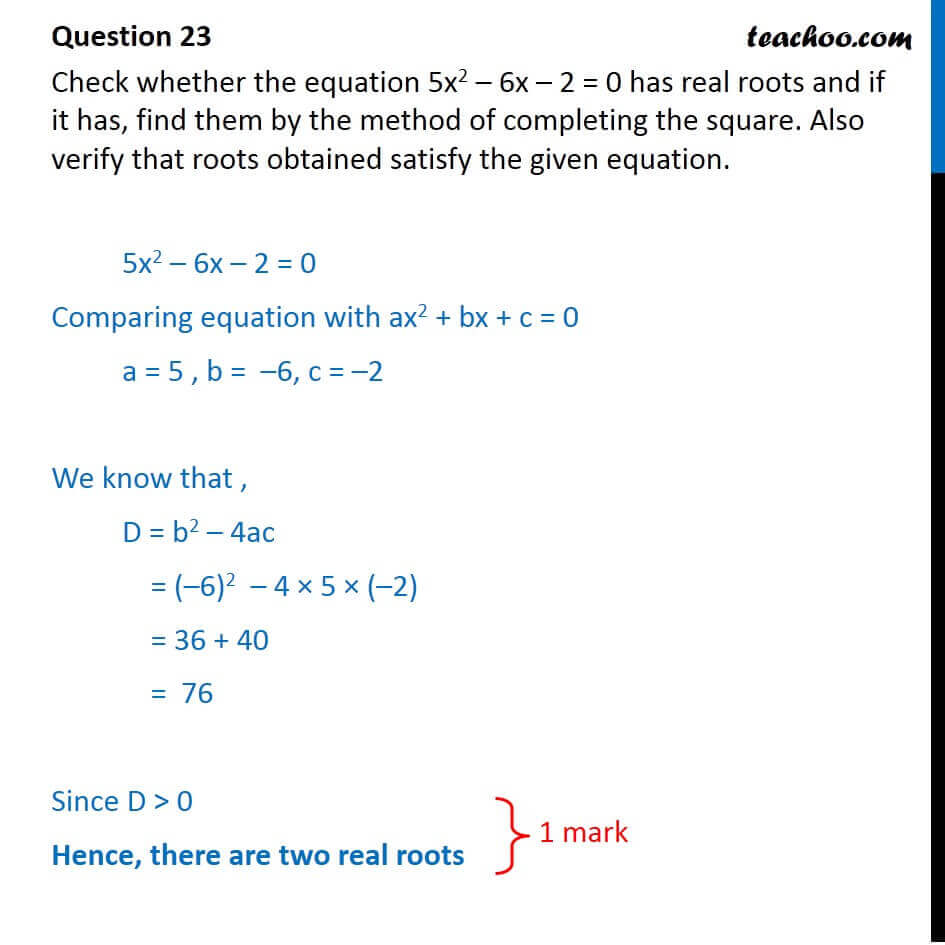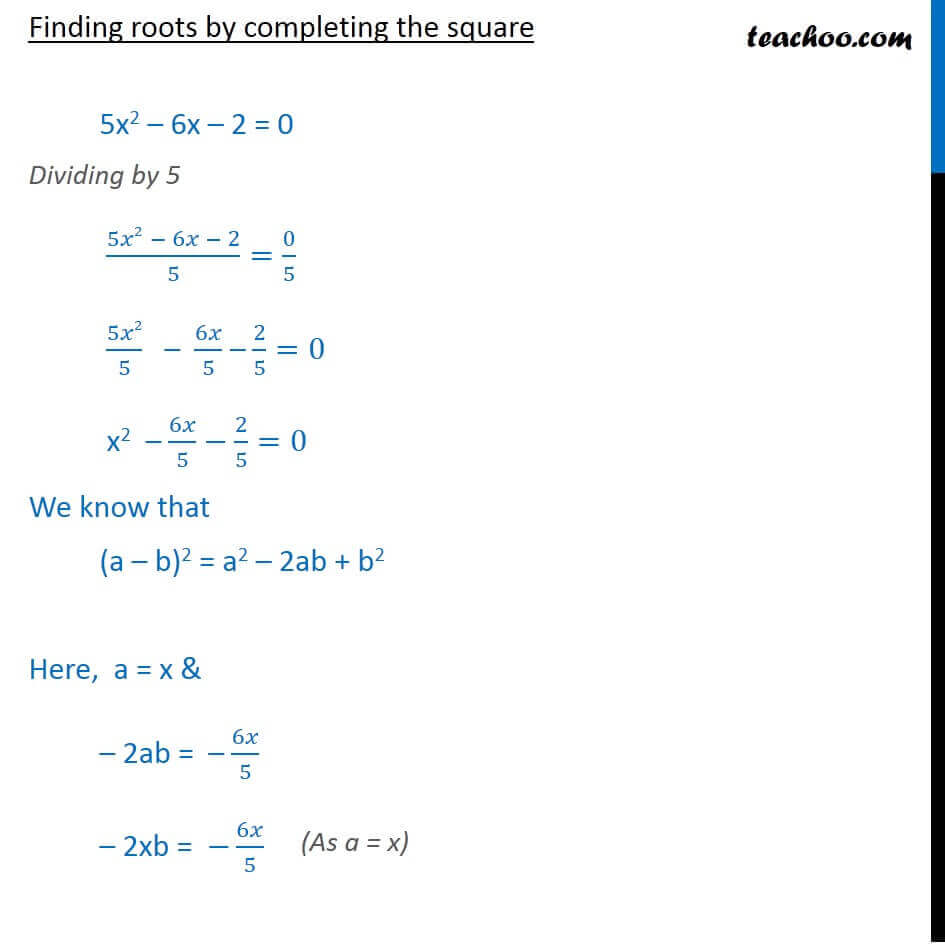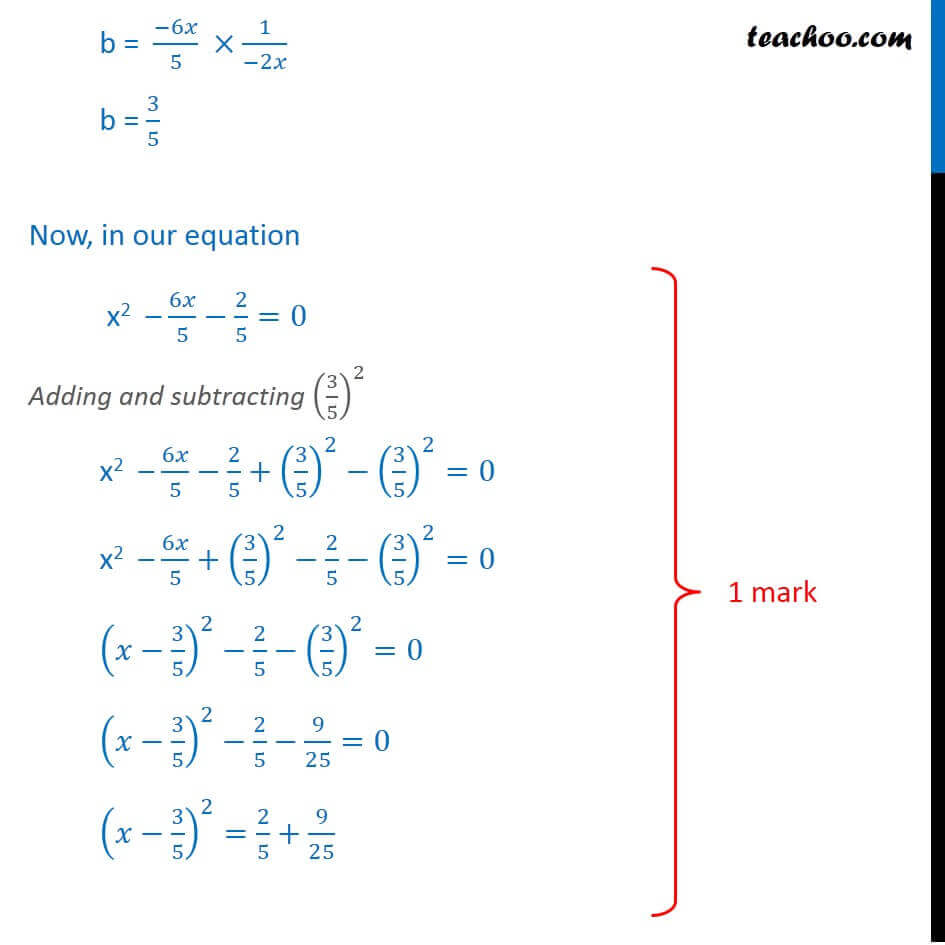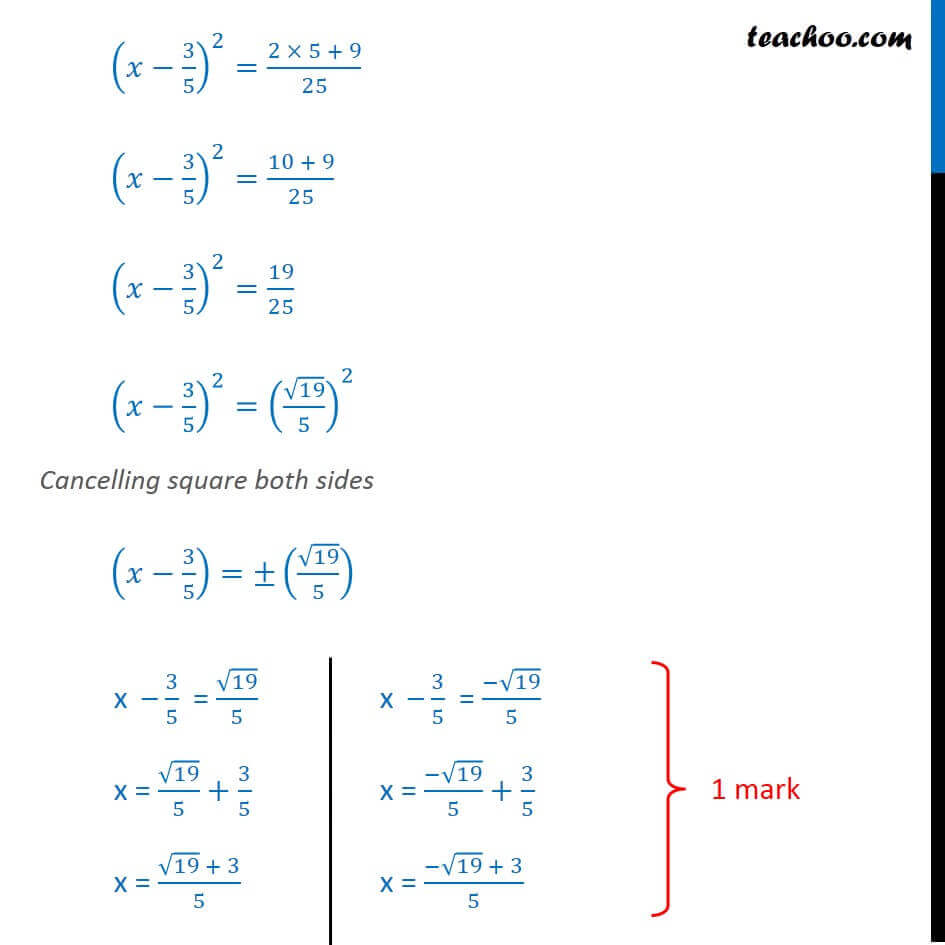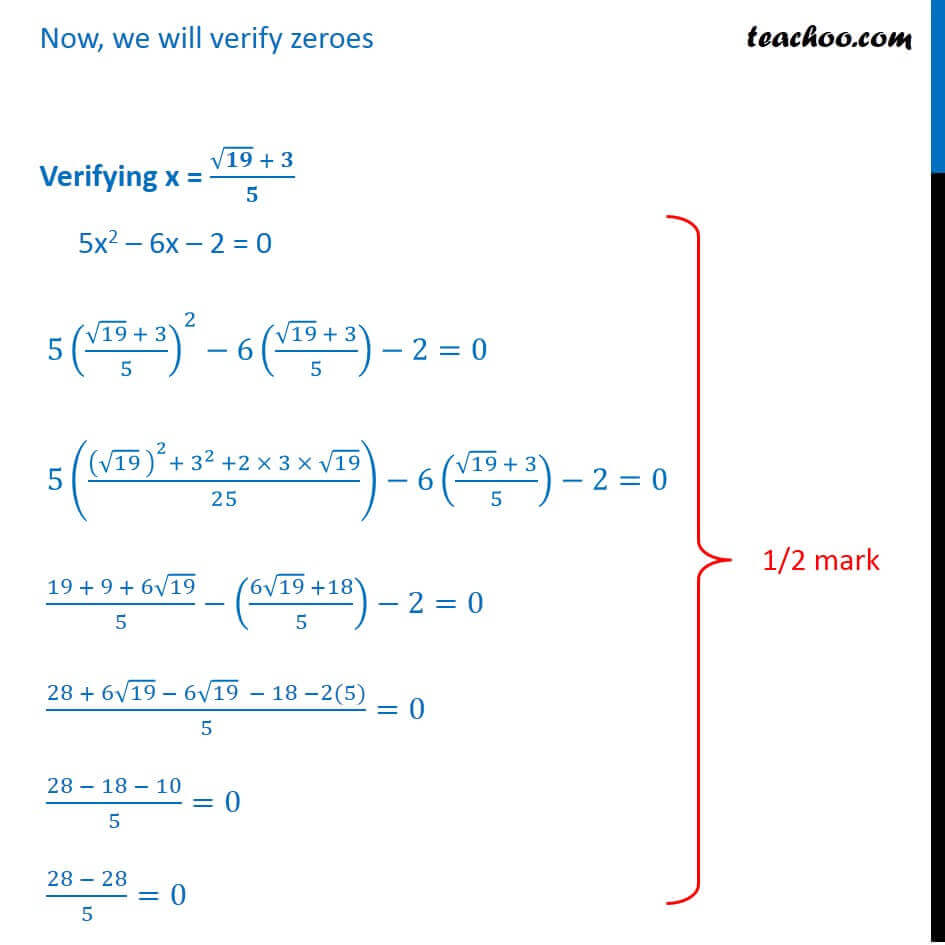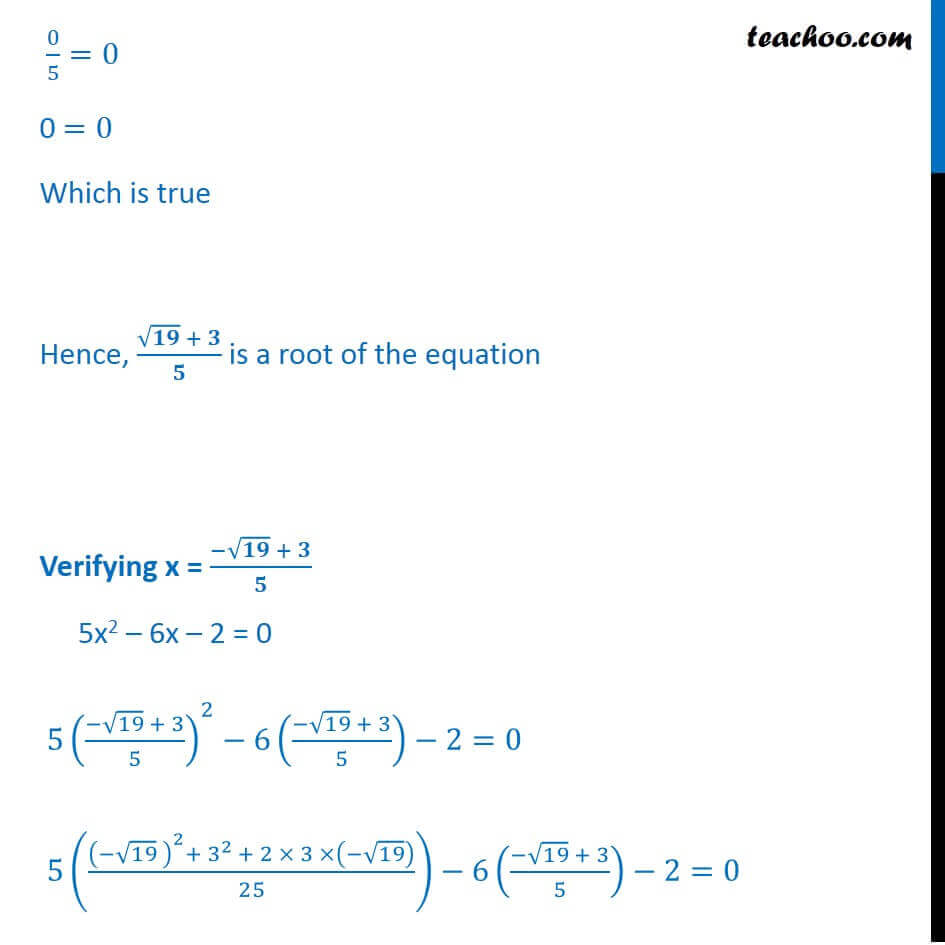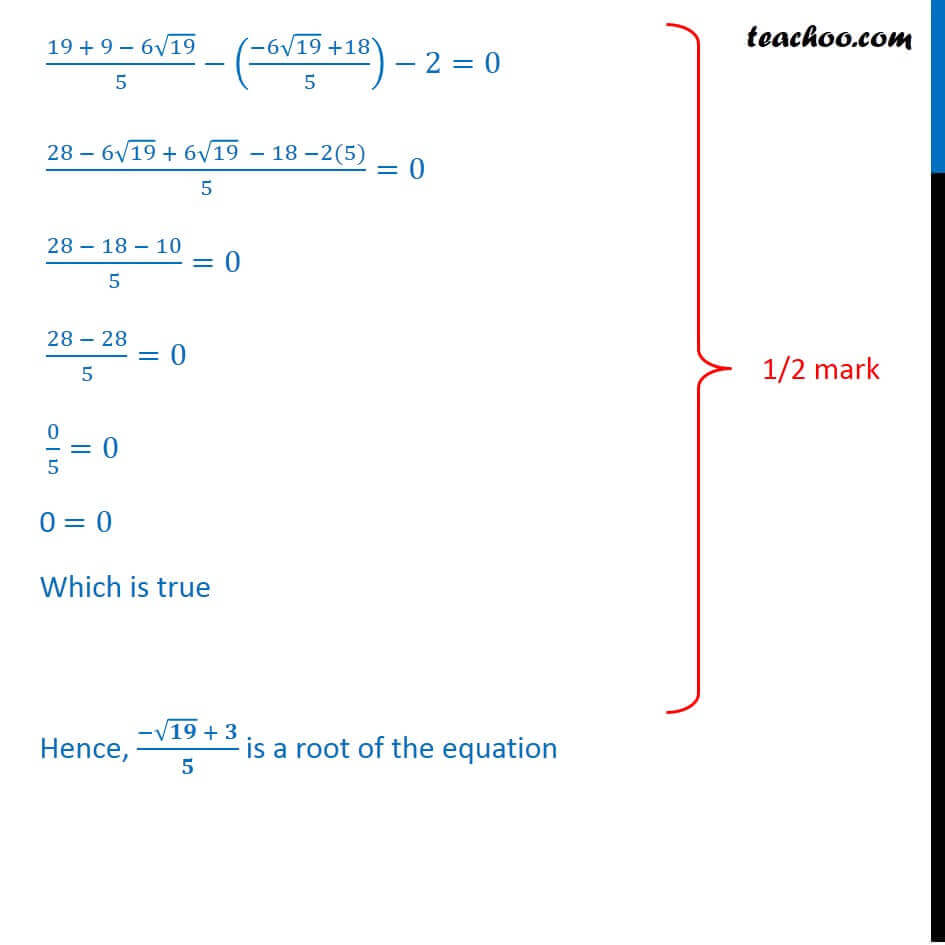Introducing your new favourite teacher - Teachoo Black, at only ₹83 per month

### Transcript

Question 23 Check whether the equation 5x2 6x 2 = 0 has real roots and if it has, find them by the method of completing the square. Also verify that roots obtained satisfy the given equation. 5x2 6x 2 = 0 Comparing equation with ax2 + bx + c = 0 a = 5 , b = 6, c = 2 We know that , D = b2 4ac = ( 6)2 4 5 ( 2) = 36 + 40 = 76 Since D > 0 Hence, there are two real roots Finding roots by completing the square 5x2 6x 2 = 0 Dividing by 5 (5 2 6 2)/5=0/5 5 2/5 6 /5 2/5=0 x2 6 /5 2/5=0 We know that (a b)2 = a2 2ab + b2 Here, a = x & 2ab = 6 /5 2xb = 6 /5 b = ( 6 )/5 1/( 2 ) b = 3/5 Now, in our equation x2 6 /5 2/5=0 Adding and subtracting (3/5)^2 x2 6 /5 2/5+(3/5)^2 (3/5)^2=0 x2 6 /5+(3/5)^2 2/5 (3/5)^2=0 ( 3/5)^2 2/5 (3/5)^2=0 ( 3/5)^2 2/5 9/25=0 ( 3/5)^2=2/5+9/25 ( 3/5)^2=(2 5 + 9)/25 ( 3/5)^2=(10 + 9)/25 ( 3/5)^2=19/25 ( 3/5)^2=( 19/5)^2 Cancelling square both sides ( 3/5)= ( 19/5) x 3/5 = 19/5 x = 19/5+3/5 x = ( 19 + 3)/5 x 3/5 = ( 19)/5 x = ( 19)/5+3/5 x = ( 19 + 3)/5 Now, we will verify zeroes Verifying x = ( + )/ 5x2 6x 2 = 0 5(( 19 + 3)/5)^2 6(( 19 + 3)/5) 2=0 5((( 19 )^2+ 3^(2 )+2 3 19)/25) 6(( 19 + 3)/5) 2=0 (19 + 9 + 6 19)/5 ((6 19 +18)/5) 2=0 (28 + 6 19 6 19 18 2(5))/5=0 (28 18 10)/5=0 (28 28)/5=0 0/5=0 0 =0 Which is true Hence, ( + )/ is a root of the equation Verifying x = ( + )/ 5x2 6x 2 = 0 5(( 19 + 3)/5)^2 6(( 19 + 3)/5) 2=0 5((( 19 )^2+ 3^(2 )+ 2 3 ( 19))/25) 6(( 19 + 3)/5) 2=0 (19 + 9 6 19)/5 (( 6 19 +18)/5) 2=0 (28 6 19 + 6 19 18 2(5))/5=0 (28 18 10)/5=0 (28 28)/5=0 0/5=0 0 =0 Which is true Hence, ( + )/ is a root of the equation